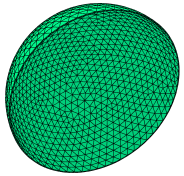﻿ Sample Problems > Usage > 3D_domains > 3d_shell

3d_shell

Navigation:  Sample Problems > Usage > 3D_domains >

3d_shell{ 3D_SHELL.PDE    This problem considers heatflow in a  spherical shell.  We solve a heatflow equation with  fixed temperatures on inner and outer  shell surfaces. }   title '3D Test - Shell'   coordinates    cartesian3   variables    udefinitions

k = 10                     { conductivity }

heat =6*k                 { internal heat source }

R1 = 1

thick = staged(0.1,0.03,0.01)

R2 = R1-thick

equations

U: div(K*grad(u)) + heat   = 0

extrusion

surface z =  -SPHERE ((0,0,0),R1)     { the bottom hemisphere }

surface z =  -SPHERE ((0,0,0),R2)

surface z = SPHERE ((0,0,0),R2)

surface z = SPHERE ((0,0,0),R1)       { the top hemisphere }

boundaries

surface 1 value(u) = 0     { fixed values on outer sphere surfaces }

surface 4 value(u) = 0

Region 1   { The outer boundary in the base projection }

layer 1 k=0.1 mesh_spacing=10*thick { force resolution of shell curve }

layer 2 k=0.1

layer 3 k=0.1 mesh_spacing=10*thick

start(R1,0)

value(u) = 0           { Fixed value on outer vertical sides }

arc(center=0,0) angle=180

natural(u)=0 line to close

Limited Region 2   { The inner cylinder shell boundary in the base projection }

surface 2 value(u) = 1   { fixed values on inner sphere surfaces }

surface 3 value(u) = 1

layer 2 void           { empty center }

start(R2,0)

arc(center=0,0) angle=180

nobc(u) line to close

monitors

grid(x,y,z)

grid(x,z) on y=0

contour(u) on x=0         { YZ plane through diameter }

contour(u) on y=0         { XZ plane through diameter }

contour(u) on z=0         { XY plane through diameter }

contour(u) on x=0.5       { YZ plane off center }

contour(u) on y=0.5       { XZ plane off center }

definitions

yp = 0.5

R0 = (R1+R2)/2

Rin = sqrt((R0-0.1)^2-yp^2)

Rout = sqrt((R0+0.1)^2-yp^2)

xin = Rin/sqrt(2)

xout = Rout/sqrt(2)

plots

grid(x,y,z)

grid(x,z) on y=0

grid(x,z) on y=yp

contour(u) on x=0         as "Temp on YZ plane through diameter"

contour(u) on y=0         as "Temp on XZ plane through diameter"

contour(u) on z=0         as "Temp on XY plane through diameter"

contour(u) on z=0.001         as "Temp on XY plane through diameter"

contour(u) on x=0.5       as "Temp on YZ plane off center"

contour(u) on y=0.5       as "Temp on XZ plane off center" report(Rin,Rout,xin,xout)